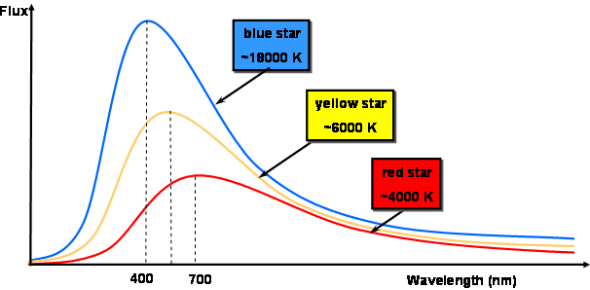# Blackbody Radiation Questions: Quiz!

10 Questions | Total Attempts: 1571SettingsCreate your own QuizDo you know about blackbody radiations? Take this blackbody radiation quiz and see how well you know this topic! In these questions, you will use the Blackbody Spectrum Simulation to investigate how the spectrum of electromagnetic radiation emitted by objects is affected by the object's temperature. In this simulation, you can input the temperature and observe the spectrum of the radiation emitted. Let's try it out. Attempt all the questions, and see how much you score. All the best for a perfect score!

• 1.
The temperature of stars in the universe varies with the type of star and the age of the star, among other things. By looking at the shape of the spectrum of light emitted by a star, we can tell something about its average surface temperature. If we observe a star's spectrum and find that the peak power occurs at the border between red and infrared light, what is the approximate surface temperature of the star? (in degrees C)
• A.

200

• B.

2000

• C.

4000

• D.

8000

• 2.
If we observe a star's spectrum and find that the peak power occurs at the border between blue and ultraviolet light, what is the surface temperature of the star? (in degrees C)
• A.

200

• B.

2000

• C.

3000

• D.

7000

• 3.
Light bulbs operate at 2500 degrees C.  What is the wavelength at which the most power is emitted for a light bulb operating at 2500 C?
• A.

200 nm

• B.

600 nm

• C.

1200 nm

• D.

6000 nm

• 4.
Is the most powerful (peak) wavelength emitted from a light bulb in the visible light range?
• A.

Yes

• B.

No

• C.

It's on the border

• 5.
Investigate how the observed spectrum responds to changing temperature. Note you are only able to change temperature here but should consider how the spectrum (power vs. color) would change if you varied other characteristics of the object. If the only change you make is to decrease the temperature of an object, the amount of power emitted at 1000 nm will increase in some cases.
• A.

True

• B.

False

• 6.
If the only change you make is to decrease the temperature of an object, the total amount of power emitted decreases in all cases.
• A.

True

• B.

False

• 7.
What is the approximate peak wavelength for a bulb operating at 2000 degrees C?
• A.

200 nm

• B.

400 nm

• C.

800 nm

• D.

1200 nm

• E.

1400 nm

• 8.
At what temperature is the most efficient temperature, in Celsius for visible light?
• A.

1000

• B.

2250

• C.

3500

• D.

4500

• E.

5500

• 9.
Use the Spectrum Simulation to investigate changes in the amount of light at visible wavelengths due to this change in temperature from 2500 C to 2000 C. What is the approximate ratio between the powers emitted at 500 nm at 2000 degrees C to that at 2500 degrees C, that is, the power emitted at 500 nm at 2000 degrees C divided by the amount of power at 500 nm at degrees 2500 C?
• A.

2 to 1

• B.

5 to 1

• C.

11 to 1

• D.

100 to 1

• 10.
Evolution is about the most efficient species for the area that will survive, so most life on the earth sees in the same visual spectrum.  If life was to develop in a solar system with a red giant (3000 C)  instead of a yellow star (5700 C)
• A.

There would be no difference in the visual light they would see.

• B.

They would see more into the ultraviolet.

• C.

They would see more in the infrared.

• D.

None of the above

Related TopicsBack to top
×

Wait!
Here's an interesting quiz for you.